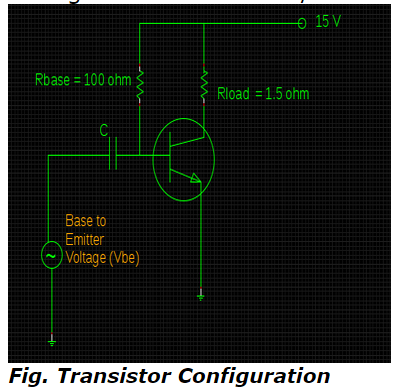# AC / DC Analysis of Transistor - MCQs with answers

## AC / DC Analysis of Transistor - MCQs with answers

Q1. Which device plays a significant role in connection of ac source without affecting or causing any sort of perturbation to dc biasing while applying AC to DC biased transistor?

a. Inductor
b. Resistor
c. Coupling Capacitor
d. Filter

Q2. What is the phase angle difference between ac output and input voltages corresponding to AC/ DC analysis of transistor?

a. 90 ° in phase with each other
b. 90 ° out-of -phase with each other
c. 180 ° in phase with each other
d. 180 ° out-of-phase with each other

ANSWER: d. 180 ° out-of-phase with each other

Q3. Compute the value of VCE for the following circuit by taking into consideration the standard values of Av = β = 100 along with peak input signal voltage of about 0.03Va. 3.75 A
b. 5.75 A
c. 3.25 A
d. 5.25 A